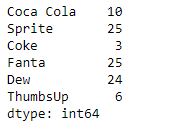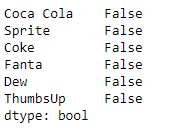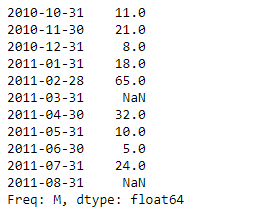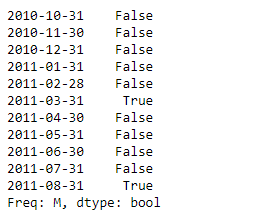# Python | Pandas Series.isnull()

Pandas series is a One-dimensional ndarray with axis labels. The labels need not be unique but must be a hashable type. The object supports both integer and label-based indexing and provides a host of methods for performing operations involving the index.

Pandas` Series.isnull()` function detect missing values in the given series object. It return a boolean same-sized object indicating if the values are NA. Missing values gets mapped to `True` and non-missing value gets mapped to `False`.

Syntax: Series.isnull()

Parameter : None

Returns : boolean

Example #1: Use `Series.isnull()` function to detect missing values in the given series object.

 `# importing pandas as pd ` `import` `pandas as pd ` ` `  `# Creating the Series ` `sr ``=` `pd.Series([``10``, ``25``, ``3``, ``25``, ``24``, ``6``]) ` ` `  `# Create the Index ` `index_ ``=` `[``'Coca Cola'``, ``'Sprite'``, ``'Coke'``, ``'Fanta'``, ``'Dew'``, ``'ThumbsUp'``] ` ` `  `# set the index ` `sr.index ``=` `index_ ` ` `  `# Print the series ` `print``(sr) `

Output :Now we will use `Series.isnull()` function to detect all the missing values in the given series object.

 `# detect missing values ` `result ``=` `sr.isnull() ` ` `  `# Print the result ` `print``(result) `

Output :As we can see in the output, the `Series.isnull()` function has returned an object containing boolean values. All values have been mapped to `False` because there is no missing value in the given series object.

Example #2 : Use `Series.isnull()` function to detect missing values in the given series object.

 `# importing pandas as pd ` `import` `pandas as pd ` ` `  `# Creating the Series ` `sr ``=` `pd.Series([``11``, ``21``, ``8``, ``18``, ``65``, ``None``, ``32``, ``10``, ``5``, ``24``, ``None``]) ` ` `  `# Create the Index ` `index_ ``=` `pd.date_range(``'2010-10-09'``, periods ``=` `11``, freq ``=``'M'``) ` ` `  `# set the index ` `sr.index ``=` `index_ ` ` `  `# Print the series ` `print``(sr) `

Output :Now we will use `Series.isnull()` function to detect all the missing values in the given series object.

 `# detect missing values ` `result ``=` `sr.isnull() ` ` `  `# Print the result ` `print``(result) `

Output :As we can see in the output, the `Series.isnull()` function has returned an object containing boolean values. All missing values have been mapped to `True`.

My Personal Notes arrow_drop_upCheck out this Author's contributed articles.

If you like GeeksforGeeks and would like to contribute, you can also write an article using contribute.geeksforgeeks.org or mail your article to contribute@geeksforgeeks.org. See your article appearing on the GeeksforGeeks main page and help other Geeks.

Please Improve this article if you find anything incorrect by clicking on the "Improve Article" button below.

Article Tags :

Be the First to upvote.

Please write to us at contribute@geeksforgeeks.org to report any issue with the above content.